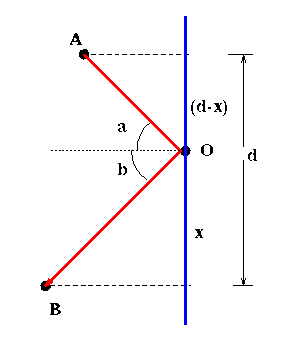# How does light travel?

Understanding how and where a rainbow appears is tied to understanding how light travels. In this section we will investigate some of the basic theories about the nature of light.

To help with geometric understanding, we will assume that light travels in rays. We begin with light rays moving through the air at a constant speed and consider the reflection of light. In 1657 the mathematician Pierre de Fermat postulated a simple principle:

Light follows a path that minimizes total travel time.## Question 1

Using Fermat's Principle, Figure 1, and calculus, determine the relationship between the incoming and outgoing angles.
• Write down an expression for the total distance that light travels between A and B, in terms of the unknown position x.
• If we assume that the speed of light through air is a constant, then distance light travels equals its speed times the time it takes to travel the distance. Use calculus and Fermat's Principle to determine an equation that x must satisfy in order to minimize the time traveled between A and B. (You do not need to solve this equation)
• Express the sines of angles a and b in terms of the side lengths of the triangles in Figure 1.
• Combine the answers to the previous two parts to determine how sin(a) and sin(b) are related when x is chosen so as to satisfy Fermat's Principle.
• Argue that this implies that a=b.

Thus you have discovered a relationship between the angles formed by incoming and outgoing rays of reflected light. We call a the angle of incidence and b the angle of reflection. In the last part of Question 1 you deduced the Law of Reflection: the angle of incidence is equal to the angle of reflection. It is also possible to demonstrate the Law of Reflection completely geometrically.
Next:Refraction
Previous:Objectives